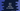# Java program to find the factors of a number## Java program to find the factors of a number:

In this post, we will learn how to find the factors of a number in Java. A number is called factor of a different number, if it can divide the number completely i.e. if the remainder is 0.

For example, 1, 2, 4 are factors of 4.

To solve this program programmatically, we can use a loop that will check all numbers from 1 to that number.

We can use any loop. In this post, I will show you how to do that using a for loop, while loop, do while loop and also by using a separate function.

### Method 1: Find all factors of a number using a for loop in Java:

Let’s take a look at the below program:

``````import java.util.ArrayList;
import java.util.List;
import java.util.Scanner;

public class Main {

public static void main(String[] args) {
Scanner sc = new Scanner(System.in);
System.out.println("Enter a number : ");

int no = sc.nextInt();
List<Integer> factorsList = new ArrayList<>();

System.out.println("Factors are : ");

for (int i = 1; i <= no; i++) {
if (no % i == 0) {
}
}

System.out.println(factorsList);
}
}``````

### Explanation:

Here,

• We are asking the user to enter a number and storing that value in the no variable.
• factorsList is an ArrayList that can hold integers. This is used to store the factors.
• Using a for loop, we are iterating from i = 1 to i = no and for each value of i, we are checking if it can perfectly divide no or not.
• If yes, we are adding that to the list factorsList.
• Once the for loop ends, we are printing the factorsList which holds all factors of no.

### Sample output:

It will print output as like below:

``````Enter a number :
6
Factors are :
[1, 2, 3, 6]

Enter a number :
7
Factors are :
[1, 7]``````

### Method 2: Find all factors of a number using a while loop in Java:

Let’s write the same program using while.

``````import java.util.ArrayList;
import java.util.List;
import java.util.Scanner;

public class Main {

public static void main(String[] args) {
Scanner sc = new Scanner(System.in);
System.out.println("Enter a number : ");

int no = sc.nextInt();
List<Integer> factorsList = new ArrayList<>();

System.out.println("Factors are : ");

int i = 1;

while(i <= no) {
if (no % i == 0) {
}
i++;
}

System.out.println(factorsList);
}
}``````

Here,

• we are using a while loop that replaces for loop.
• In this loop, the while loop runs from i = 1 to i = no. For each iteration, we are incrementing the value of i by 1. Also, similar to the above program, we are adding the value of i to factorsList if i is a factor of no.
• Finally we are printing the value of factorsList.

### Method 3: Find all factors of a number using a do-while loop in Java:

We can also use a do-while loop to find the factors. For example:

``````import java.util.ArrayList;
import java.util.List;
import java.util.Scanner;

public class Main {

public static void main(String[] args) {
Scanner sc = new Scanner(System.in);
System.out.println("Enter a number : ");

int no = sc.nextInt();
List<Integer> factorsList = new ArrayList<>();

System.out.println("Factors are : ");

int i = 1;

do {
if (no % i == 0) {
}
i++;
}while(i <= no);

System.out.println(factorsList);
}
}``````

It will print the same output.

### Method 4: Find all factors of a number using a different function:

We can also use a different function and put the part to calculate the factors in that function. Using a function has many advantages like we can remove reusable code and put it in a function.

For example, the below program uses the findFactors function to get the list of factors. I am using do-while, but you can use any other loop if you want. It returns a list of integers holding the factors.

``````import java.util.ArrayList;
import java.util.List;
import java.util.Scanner;

public class Main {
private static List<Integer> findFactors(int no) {
List<Integer> factorsList = new ArrayList<>();
int i = 1;
do {
if (no % i == 0) {
}
i++;
} while (i <= no);
return factorsList;
}

public static void main(String[] args) {
Scanner sc = new Scanner(System.in);

System.out.println("Enter a number : ");
int no = sc.nextInt();

System.out.println("Factors are : ");
System.out.println(findFactors(no));
}
}``````

findFactors is a private function, but we can make it public and call it from different parts of the program.